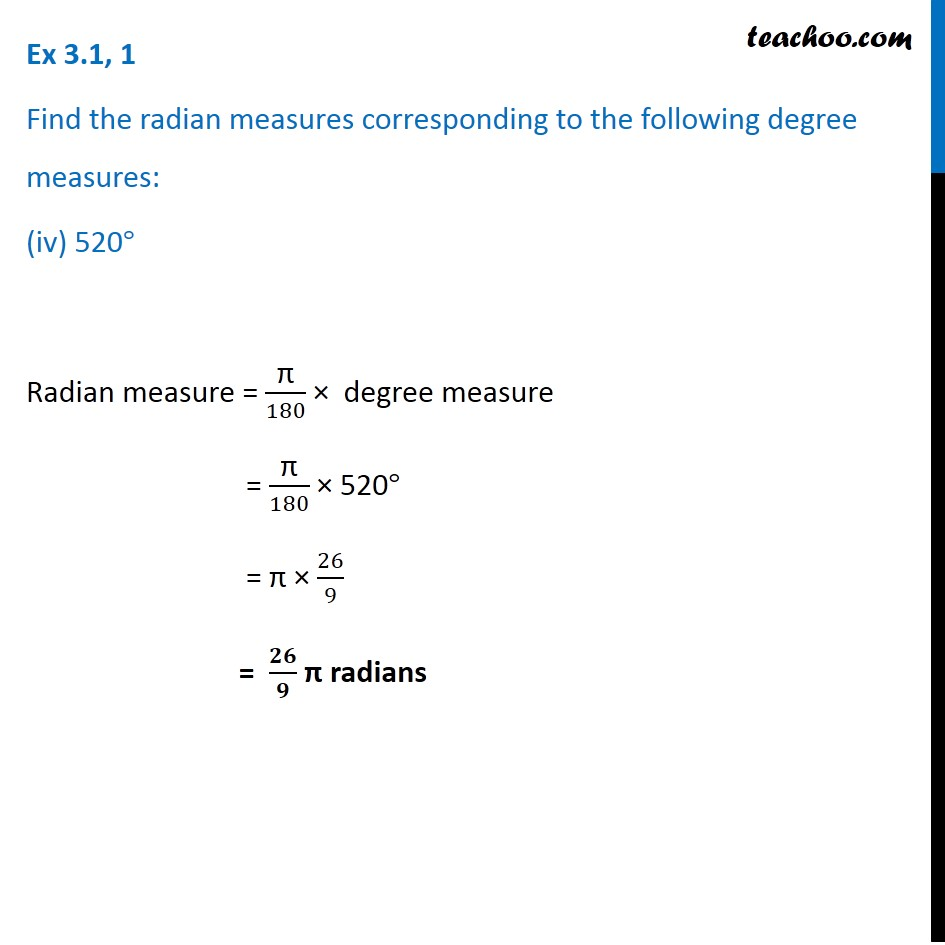1. Chapter 3 Class 11 Trigonometric Functions (Term 2)
2. Serial order wise
3. Ex 3.1

Transcript

Ex 3.1, 1 Find the radian measures corresponding to the following degree measures: (iv) 520° Radian measure = "π" /180 × degree measure = "π" /180 × 520° = "π" × 26/9 = 𝟐𝟔/𝟗 "π" radians